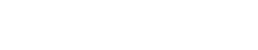## Questions

1. When spraying a field of water using a hose pipe, it is common to reduce the pipes opening in order to spray water furthest. Other than pressure, what other quality is varied in the process?
2. The figure below shows water forces through a hydraulic system by a pump. An air chamber is used to maintain a continuous flow of water during both the up stroke and down stroke of the piston pump.Explain how the continuous flow of water is maintained.
3. A pupil blows a current of air over the surface of a sheet of paper held close to its mouth. State and explain what happens to the paper.
4. Two table Tennis balls are in the same level while suspended from threads a short distance apart. A stream of air is blown between the balls in a horizontal direction. Explain what happens to the balls
5. The figure represents a tube through which liquid is flowing in the direction shown by the arrow. Copy the diagram and show on it the relative positions of the level of the liquid in sections marked, X, Y and Z.6. State how the pressure in a moving fluid varies with speed of the water is steadily increased from low to high value.
7. Water flows in a horizontal smooth pipe. State the changes that would be observed in the nature of the flow if the speed of the water is steadily increased from low to high value?
8. The figure below shows a pith ball in a flask. When a jet of air is blown over the mouth of the flask as shown, the pith ball is observed to rise from the bottom.Explain the observation
9. State Bernoulli’s principle. (1 mark)
10. Figure 2 shows a tube of varying cross sectional area. VVV3 and V4 represents the speeds of water as it flows steadily though the sections of the tube.Arrange the speeds V1, V2, V3 and V4 in decreasing order starting with the highest.
11. Water flows along a horizontal pipe of cross sectional area 30cm2. The speed of the water is 4 m/s but it reaches 7.5 m/s in a constriction in the pipe. Calculate the area of the constriction.
12. A heart pumps blood at a rate of 1.8 x 103 cm3/min though its aorta is of cross sectional area 0.6 cm3. The blood spreads into a capillary network that is equivalent to about 4 x 106 fine tubes each of diameter 6 x 10-3 cm.
Calculate:
1. The average velocity of blood in the aorta
2. The average blood velocity in the capillary tubes.

1. Velocity
2. Forward (upstroke) - The valve opens, pressure forces water flow into the air chamber and air is compressed. Backward (down stroke) - valve closes; compressed air expands hence continuous flow of water.
3. The paper rises up because the pressure above becomes less than the pressure below.
4. Balls move towards each other; pressure in the space between them decreases to below that on the outside.
5. hx=hz>hy
6. Pressure is inversely proportional to the speed; or speed increases as pressure decreases.
7. At low speeds the speed is streamlined while at high speeds, the flow is turbulent.
8. Air over the mouth of the bottle moves faster than air under.
Pressure above the mouth of the bottle is less than pressure inside the bottle (hence dynamic lift of ball)
9. At steady rate the sum of the pressure, the potential energy per unit volume and kinetic energy per unit volume in fluid is a constant
Provided a finish is non-viscous, incompressible and its flow streamline and increase in its velocity produces a corresponding decrease in pressure.
When the speed of a fluid increases, the pressure in the fluid decreases and vice versa.
10. V2, V4, V1, V3
11. A1V1 = A2V2
30 x 4 = 7.5 x A2
A2= 16 cm2
12.
1. 50 (cm/s)
2. 26.53 (cm/s)

• ✔ To read offline at any time.
• ✔ To Print at your convenience
• ✔ Share Easily with Friends / Students